Tamilnadu State Board New Syllabus Samacheer Kalvi 8th Maths Guide Pdf Chapter 7 Information processing Ex 7.2 Text Book Back Questions and Answers, Notes.

## Tamilnadu Samacheer Kalvi 8th Maths Solutions Chapter 7 Information processing Ex 7.2

Question 1.
Using repeated division method, find the HCF of the following:
(i) 455 and 26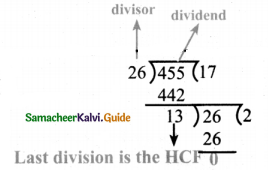Step 1: The larger number should be dividend 455 & smaller number should be divisor = 26
Step 2: After 1st division, the remainder becomes new divisor & the previous divisor becomes next dividend.
Step 3: This is done till remainder is zero.
Step 4: The last divisor is the HCF L.
∴ Ans: HCF is 13.(ii) 392 and 256
256 is smaller, so it is the 1st divisor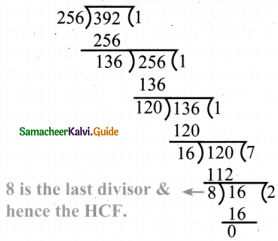∴ HCF = 8

(iii) 6765 and 610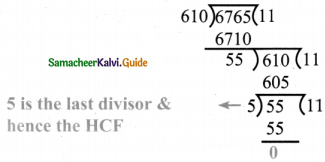∴ HCF = 5(iv) 184, 230 and 276
First let us take 184 & 230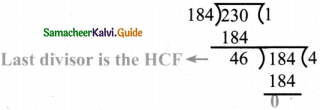∴ 46 is the HCF of 184 and 230
Now the HCF of the first two numbers is the dividend for the third number.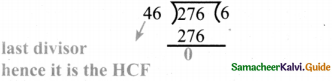∴ Ans: HCF of 184, 230 & 276 is 46

Question 2.
Using repeated subtraction method, find the HCF of the following:
(i) 42 and 70
Let number be m & n m > n
We do, m – n & the result of subtraction becomes new ‘m’. if m becomes less than n,
we do n – m and then assign the result as n. We should do this till m n. When m = n then ‘m’ is the HCF.
42 and 70
m = 70 n = 42
70 – 42 = 28

now m = 42, n = 28
42 – 28 = 14.

now m = 28, n = 14
28 – 14 = 14.

now m = 14. n = 14;
we stop here as m = n
∴ HCF of 42 & 70 is 14(ii) 36 and 80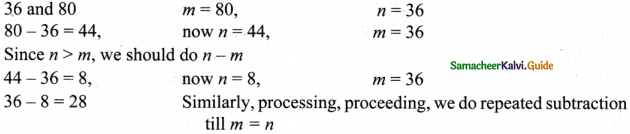28 – 8 = 20
20 – 8 = 12
12 – 8 = 4
8 = 4 = 4
now m = n = 4
∴ HCF is 4

(iii) 280 and 420
Let m = 420, n = 280
m – n = 420 – 280 = 140
now m = 280, n = 140
m – n = 280 – 140 = 140
now m = n = 140
∴ HCF is 140(iv) 1014 and 654
Let m = 1014, n = 654
m – n = 1014 – 654 = 360
now m = 654, n = 360
m – n = 654 – 360 = 294
now m = 360, n = 294
m – n = 360 – 294 = 66
now m = 294 n = 66
m – n = 294 – 66 = 228
now m = 66, n = 228
n – m = 228 – 66 = 162
now m = 162, n = 66
= 162 – 66 = 96
n – m = 96 – 66 = 30
Similarly, 66 – 30 = 36
36 – 30 = 6
30 – 6 = 24
24 – 6 = 18
18 – 6 = 12
12 – 6 = 6 now m = n
∴ HCF of 1014 and 654 is 6Question 3.
Do the given problems by repeated subtraction method and verify the result.
(i) 56 and 12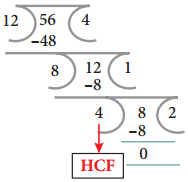Let n = 56 & n = 12
m – n = 56 – 12 = 44
now m = 44, n = 12
m – n = 44 – 12 = 32
m – n = 32 – 12 = 20
m – n = 20 – 12 = 8
n – m = 12 – 84
m – n = 8 – 4 = 4. now m = n
∴ HCF of 56 & 12 is 4(ii) 320, 120 and 95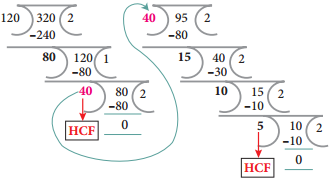Let us take 320 & 120 first m = 320, n = 120
m – n = 320 – 120 = 200
m = 200, n = 120
∴ m – n = 200 – 120 = 80
120 – 80 = 40
80 – 40 = 40
∴ m = n = 40 → HCF of 320, 120
Now let us find HCF of 40 & 95
m = 95, n = 40
∴ m – n = 95 – 40 = 55
55 – 40 = 15
40 – 15 = 25
25 – 15 = 10
15 – 10 = 5
HCF of 40 & 95 is 5
10 – 5 = 5
∴ HCF of 320 120 & 95 is 5Question 4.
Kalai wants to cut identical squares as big as she can, from a piece of paper measuring 168mm and by 196mm. What is the length of the side of the biggest square? (To find HCF using repeated subtraction method)
Sides are 168 & 196
To find HCF of 168 & 196, we are to use repeated subtraction method.
∴ m = 196, n = 168
m – n = 196 – 168 = 28

now n = 28, m = 168
m – n = 168 – 28 = 140

now m = 140, n = 28
m – n = 140 – 28 = 112

now m = 112, n = 28
m – n = 112 – 28 = 84

now m = 84, n = 28
m – n = 84 – 28 = 56

now m = 56, n = 28
m – n = 56 – 28 = 28
∴ HCF is 28
∴ Length of biggest square is 28Objective Type Questions

Question 5.
What is the eleventh Fibonacci number?
(a) 55
(b) 77
(c) 89
(d) 144
(c) 89
Hint: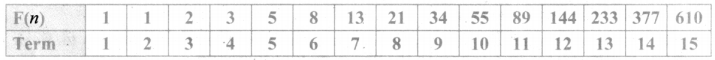∴ 11th Fibonacci number is 89

Question 6.
If F(n) is a Fibonacci number and n = 8, which of the following is true?
(a) F(8) = F(9) + F( 10)
(b) F(8) = F(7) + F(6)
(c) F(8) = F(10) × F(9)
(d) F(8) = F(7) – F(6)
(b) F(8) = F(7) + F(6)
Hint:
Given F(n) is a Fibonacci number & n = 8
∴ F(8) = F(7) + F (6) as any term in Fibonacci series is the sum of preceding 2 termsQuestion 7.
Every 3rd number of the Fibonacci sequence is a multiple of_______
(a) 2
(b) 3
(c) 5
(d) 8
(a) 2
Hint:
Every 3rd number in Fibonacci sequence is a multiple of 2

Question 8.
Every _____ number of the Fibonacci sequence is a multiple of 8
(a) 2th
(b) 4th
(c) 6th
(d) 8th
(c) 6thQuestion 9.
The difference between the 18th and 17th Fibonacci number is
(a) 233
(b) 377
(c) 610
(d) 987
(d) 987
Hint:
F(18) = F(17) + F(16)
F(18) – F(17) = F(16) = F(15) + F(14)
= 610 + 377 = 987

Question 10.
Common prime factors of 30 and 250 are
(a) 2 × 5
(b) 3 × 5
(c) 2 × 3 × 5
(d) 5 × 5
(a) 2 × 5
Prime factors of 30 are 2 × 3 × 5
Prime factors of 250 are 5 × 5 × 5 × 2
∴ Common prime factors are 2 × 5Question 11.
Common prime factors of 36,60 and 72 are
(a) 2 × 2
(b) 2 × 3
(c) 3 × 3
(d) 3 × 2 × 2
(d) 3 × 2 × 2
Hint:
Prime factors of 36 are 2 × 2 × 3 × 3
Prime factors of 60 are 2 × 2 × 3 × 5
Prime factors of 72 are 2 × 2 × 2 × 3 × 3
∴ Common prime factors are 2 × 2 × 3

Question 12.
Two numbers are said to be co-prime numbers if their HCF is
(a) 2
(b) 3
(c) 0
(d) 1